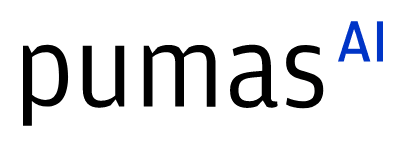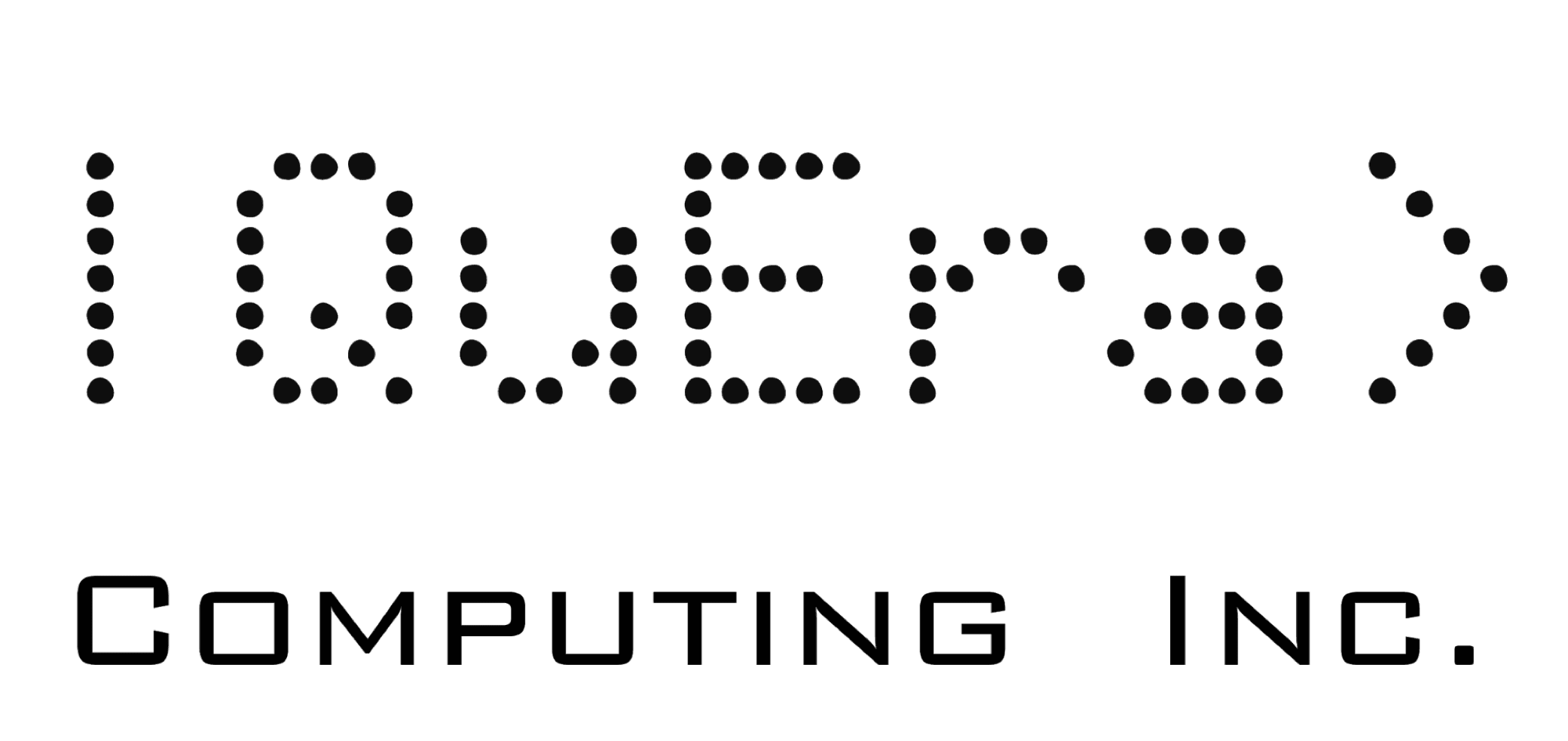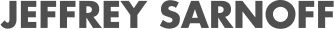# LinearSolve.jl: because A\b is not good enough

07/28/2022, 4:50 PM5:00 PM UTC
Red

#### Abstract:

Need to solve Ax=b for x? Then use A\b! Or wait, no. Don't. If you use that method, how do you swap that out for a method that performs GPU offloading? How do you switch between UMFPACK and KLU for sparse matrices? Krylov subspace methods? What does all of this mean and why is A\b not good enough? Find out all of this and more at 11. P.S. LinearSolve.jl is the answer.

#### Description:

We tell people that to solve Ax=b, you use A\b. But in reality, that is insufficient for many problems. For dense matrices, LU-factorization, QR-factorization, and SVD-factorization approaches are all possible ways to solve this, each making an engineering trade-off between performance and accuracy. While with Julia's Base you can use lu(A)\b, qr(A)\b, and svd(A)\b, this idea does not scale to all of the cases that can arise. For example, Krylov subspace methods require you set a tolerance `tol`... how do you expect to do that? krylov(A;tol=1e-7)\b? No, get outta here, the libraries don't support that. And even if they did, this still isn't as efficient as... you get the point.

This becomes a major issue with packages. Say Optim.jl uses a linear solve within its implementation of BFGS (it does). Let's say the code is A\b. Now you know in your case A is a sparse matrix which is irregular, and thus KLU is 5x faster than the UMFPACK that Julia's \ defaults to. How do you tell Optim.jl to use KLU instead? Oops, you can't. But wouldn't it be nice if you could just pass `linsolve = KLUFactorization()` and have it do that?

Okay, we can keep belaboring the point, which is that the true interface of linear solvers needs to have many features and performance, and it needs to be a multiple dispatching interface so that it can be used within other packages and have the algorithms swapped around by passing just one type. What a great time for the SciML ecosystem to swoop in! This leads us to LinearSolve.jl, a common interface for linear solver libraries. What we will discuss is the following:

• Why there are so many different linear solver methods. What are they used for? When are which ones recommended? Short list: LU, QR, SVD, RecursiveFactorization.jl (pure Julia, and the fastest?), GPU-offload LU, UMFPACK, KLU, CG, GMRES, Pardiso, ...
• How do you swap between linear solvers in the LinearSolve.jl interface. It's easy: solve(prob,UMFPACKFactorization()) vs solve(prob,KLUFactorization()).
• How do you efficiently reuse factorizations? For example, the numerical factorization stage can be reused when swapping out `b` if doing many `A\b` operations. But did know that if A is a sparse matrix you only need to perform the symbolic factorization stage once for each sparsity pattern? How do you do all of this efficiently? LinearSolve.jl has a caching interfaces that automates all of this!
• What is a preconditioner? How do you use preconditioners?

We will showcase examples where stiff differential equation solving is accelerated by over 20x just by swapping out to the correct linear solvers (https://diffeq.sciml.ai/stable/tutorials/advanced_ode_example/). This will showcase that it's not a small detail, and in fact, every library should adopt this swappable linear solver interface.## Media partners## Community partners